# 博客

## [Ynoi2015]Day2题解

2019-10-28 10:48:32 By OldDriverTree

### T1：いまこの時の輝きを（Version1）

Idea：hhf Solution：lxl Std：lxl Data：lxl

https://www.luogu.org/problem/P5071

### T2：どうか、忘れないで（Version1）

Idea：lxl Solution：lxl Std：lxl Data：lxl

https://www.luogu.org/problem/P5072

$2^{len-y}$ 是除了 $x$ 之外的数有这么多个不同的子序列，这些对 $x$ 的贡献没有影响

$2^y-1$ 是所有 $x$ 构成的子序列中有这么多种至少包含一个 $x$ ，有 $1$ 种不包含 $x$

$2^1,2^2…2^{\sqrt n} % p$

### T3：世界で一番幸せな女の子（Version2）

Idea：lxl Solution：ccz Std：lxl Data：lxl

https://www.luogu.org/problem/P5073

cur -> L()为cur节点内部的左端最大前缀和的一个分段函数

cur -> R()为cur节点内部的右端最大后缀和的一个分段函数

cur -> MID()为cur节点内部的最大子段和的一个分段函数

cur -> L(x)代表cur节点整体被加了$x$后其最大前缀和

cur -> R(x)代表cur节点整体被加了$x$后其最大后缀和

cur -> MID(x)代表cur节点整体被加了$x$后其最大子段和

cur -> L(x) = max( cur -> left -> L(x) , cur -> right -> L(x) + cur -> left -> sum );

cur -> R(x) = max( cur -> left -> R(x) + cur -> right -> sum , cur -> right -> R(x) );

cur -> MID(x) = max( cur -> left -> MID(x) , cur -> right -> MID(x) , cur -> left -> R(x) + cur -> right -> L(x) );## [Ynoi2015]Day1题解

2019-10-28 09:58:08 By OldDriverTree

### T1：ただいま帰りました（Version3）

Idea：lxl Solution：lxl Std：lxl Data：lxl

https://www.luogu.org/problem/P5068

### T2：いずれその陽は落ちるとしても（Version?）

Idea：ccz Solution：ccz Std：ccz Data：ccz

https://www.luogu.org/problem/P5069

### T3：たとえ未来が見えなくても（Version3）

Idea：lxl Solution：lxl Std：lxl Data：lxl

https://www.luogu.org/problem/P5070## [Ynoi2014]Day2题解

2019-10-27 11:52:44 By OldDriverTree

### T1：帰らぬ者と、待ち続けた者たち（Version?）

Idea：kcz Solution：kcz/lca Std：kcz/lca Data：kcz

https://www.luogu.org/problem/P5065

$k$取$\sqrt n$时，

### T2：誰も彼もが、正義の名のもとに（Version5）

Idea：lxl Solution：lxl Std：lxl Data：lxl

https://www.luogu.org/problem/P5066

### T3：消えない過去、消えていく未来（Version?）

Idea：mcfx Solution：mcfx Std：mcfx Data：mcfx

https://www.luogu.org/problem/P5067

+ 和 - 节点由于运算优先级相同，可以一起处理。

1.加入一个括号；

2.删除一对括号；

3.加入或删除一个其他节点。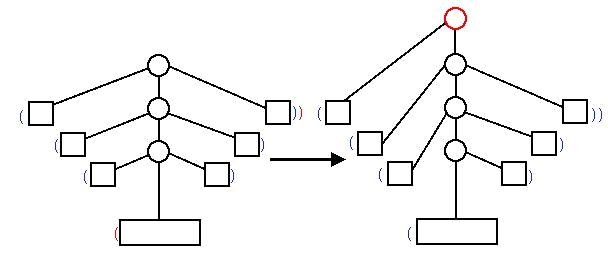## [Ynoi2014]Day1题解

2019-10-19 02:35:57 By OldDriverTree

### T1：太陽の傾いたこの世界で（Version1）

Idea：ccz Solution：ccz Std：ccz Data：ccz

https://www.luogu.org/problem/P5062

### T2：空の上の森の中の（Version1）

Idea：zcy Solution：lxl Std：lxl Data：lxl

https://www.luogu.org/problem/P5063

1.区间加

2.单点修改

3.区间rank

$O( m \sqrt n + m \sqrt {n/2} + m \sqrt {n/4} + ... )$ = $O( m \sqrt n )$

### T3：この戦いが終わったら（Version3）

https://www.luogu.org/problem/P5064## [Ynoi2018]Day2题解

2019-09-16 12:24:16 By OldDriverTree

### T1：そらのおとしもの（Version2）

Idea：lxl Solution：lxl Std：lxl Data：lxl

https://www.luogu.org/problem/P5397

http://www.51nod.com/Challenge/Problem.html#problemId=2046

"弑尽破净的第四分块" （妈呀这名字好中二啊（谁叫我要用日本轻小说中的东西命名真是作死））

$O( n \sqrt n + m \sqrt n )$

x取遍所有值，y只有所有大的值，总$O( \sqrt n )$个### T2：ゴシック（Version2）

Idea：lxl Solution：lxl Std：lxl Data：lxl

https://www.luogu.org/problem/P5398

https://cometoj.com/problem/1072

"点缀光辉的第十四分块" （妈呀这名字好中二啊（谁叫我要用日本轻小说中的东西命名真是作死））

$[l1,r1]$区间的答案为红色部分的贡献，$[l1,r2]$区间的答案为红色部分加上绿色部分的贡献，$r2$的前缀贡献为所有的贡献，$r1$的前缀贡献为红色的贡献与前两个蓝色的贡献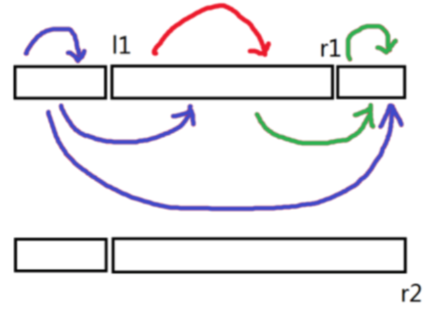$[l,r]$中$x$的倍数个数：每次前缀加入一个数$a_i$的时候，我们把$a_i$的约数位置所对应的数组$pre1$的位置都++

$[l,r]$中$x$的约数个数：这里考虑对$x$的约数进行一次根号分治### T3：駄作（Version1）

Idea：lxl Solution：ccz Std：ccz Data：ccz

https://www.luogu.org/problem/P5399

https://loj.ac/problem/6612

"？？？？的第七分块" （不中二了）

1.每个块是树

2.每个块中有两个特殊的点，称为端点 1 和端点 2。

3.不同块的边集不相交

4.一个块中的顶点，除端点外，其余顶点不在其它块中出现

5.如果一个顶点在多个块中出现，那么它一定是某一个块的端点 2，同时是其余包含这个顶点的块的端点 1

6.如果把所有块的端点作为点，每块的端点 1 和端点 2 连有向边，则得到一棵有根树## [Ynoi2018]Day1题解

2018-12-12 16:09:11 By OldDriverTree

### T1：いろとりどりのセカイ（Version3）

Idea：lxl Solution：lxl Std：lxl Data：lxl

https://www.luogu.org/problemnew/solution/P4117

https://www.lydsy.com/JudgeOnline/problem.php?id=5143

http://codeforces.com/problemset/problem/896/E

"突刺贯穿的第二分块" （妈呀这名字好中二啊（谁叫我要用日本轻小说中的东西命名真是作死））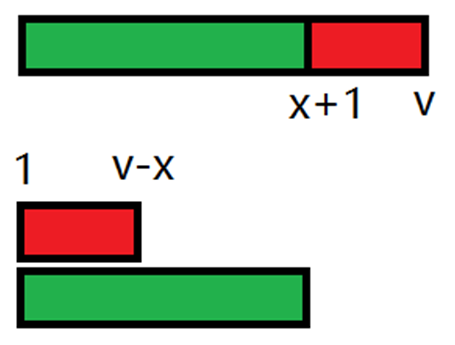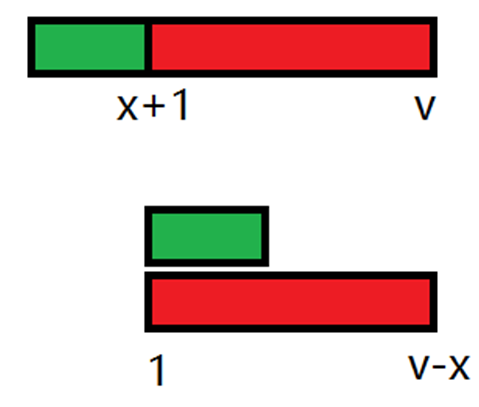$O( 1 )$把块内值为x的全部变成$x+v$

$O( \sqrt n )$求出块内经过大量操作后，每个数的值

1.定义f[i][j]为第i块里面所有值为j的数的下标的链表

2.可以每块每个值维护一个vector

3.可以用一个并查集维护

1. merge( x , y )

2. for( i = 1 ; i <= sqrtn ; i++ ) find( i )### T2：終末なにしてますか？忙しいですか？救ってもらっていいですか？（Version6）

Idea：lxl&zyz Solution：ccz Std：lxl Data：lxl

https://www.luogu.org/problemnew/show/P4680

https://www.lydsy.com/JudgeOnline/problem.php?id=5144

"深浅值藏的第六分块" （妈呀这名字好中二啊（谁叫我要用日本轻小说中的东西命名真是作死））

https://www.lydsy.com/JudgeOnline/problem.php?id=5089

cur -> L()为cur节点内部的左端最大前缀和的一个分段函数

cur -> R()为cur节点内部的右端最大后缀和的一个分段函数

cur -> MID()为cur节点内部的最大子段和的一个分段函数

cur -> L(x)代表cur节点整体被加了x后其最大前缀和

cur -> R(x)代表cur节点整体被加了x后其最大后缀和

cur -> MID(x)代表cur节点整体被加了x后其最大子段和

cur -> L(x) = max( cur -> left -> L(x) , cur -> right -> L(x) + cur -> left -> sum );

cur -> R(x) = max( cur -> left -> R(x) + cur -> right -> sum , cur -> right -> R(x) );

cur -> MID(x) = max( cur -> left -> MID(x) , cur -> right -> MID(x) , cur -> left -> R(x) + cur -> right -> L(x) );

P.S这题我写的极其恶心写了一周（虽然就是每天逃几节课来写的）### T3：未来日記（Version2）

Idea：fdy Solution：fdy&lxl Std：lxl&csy Data：lxl

https://www.luogu.org/problemnew/show/P4119

https://www.lydsy.com/JudgeOnline/problem.php?id=5145

http://acm.hdu.edu.cn/showproblem.php?pid=6079

"望月悲叹的最初分块" （妈呀这名字好中二啊（谁叫我要用日本轻小说中的东西命名真是作死））## [Ynoi2016]Day2题解

2018-12-11 12:14:12 By OldDriverTree

### T1：JabberWocky

Idea：lxl Solution：lxl Std：lxl Data：lxl&zcy

https://www.luogu.org/problemnew/show/P4118

https://www.lydsy.com/JudgeOnline/problem.php?id=5394

$a^x\;mod\; p\;=\;a^{x\;mod\;Φ(p)+(x>Φ(p)?Φ(p):0)}\;mod\;p$

### T2：Which Dreamed It

Idea：lxl Solution：lxl Std：lxl Data：lxl

https://www.luogu.org/problemnew/show/P4692

https://www.lydsy.com/JudgeOnline/problem.php?id=5395

### T3：JabberWockyII

Idea：ccz Solution：ccz Std：ccz Data：ccz

https://www.luogu.org/problemnew/show/P4693

https://www.lydsy.com/JudgeOnline/problem.php?id=5396

1.竖直路径表示一个点序列，每个点是上一个点的孩子；

2.水平路径表示一个点序列，这些点的父亲相同，每个点是上一个点右边的第一个点；

3.树上每个点在且只在一条路径中出现；

4.对每个点，它的孩子可以被表示成0到2条水平路径，除了至多一个点在某条竖直路径上。

A和B是把一条路径从水平变为竖直或从竖直变为水平，可以打标记实现，注意维护4条性质

C为换根，竖直访问然后打上翻转等标记，需要注意对孩子顺序的规定

D和E只需进行水平/竖直访问然后进行普通的区间修改查询

F为初始化，略## [Ynoi2016]Day1题解

2018-12-10 13:51:39 By OldDriverTree

### T1：Down The Rabbit Hole

Idea：lxl Solution：lxl Std：lxl Data：lxl

https://www.luogu.org/problemnew/show/P4688

https://www.lydsy.com/JudgeOnline/problem.php?id=4939

https://loj.ac/problem/6199

y+w…y+z-1这些位置填上0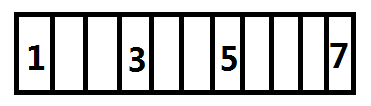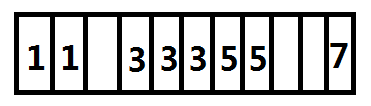### T2：It's My Own Invention

Idea：lxl改编 Solution：lxl Std：lxl Data：lxl

https://www.luogu.org/problemnew/show/P4689

https://www.lydsy.com/JudgeOnline/problem.php?id=4940

https://loj.ac/problem/6200

F(l1,r1,l2,r2)=F(1,r1,1,r2)-F(1,l1-1,1,r2)-F(1,r1,1,l2-1)+F(1,l1-1,1,l2-1)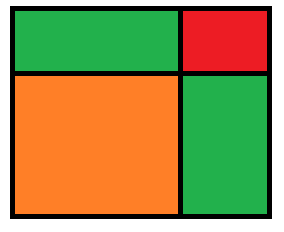### T3：Looking Glass Insect

Idea：lxl Solution：lxl Std：lxl Data：lxl

https://www.luogu.org/problemnew/solution/P4690

https://www.lydsy.com/JudgeOnline/problem.php?id=4941

https://loj.ac/problem/6201## 三道经典分块题的更优复杂度解法&[Ynoi2019模拟赛]题解

2018-11-20 19:52:35 By OldDriverTree

1.强制在线的区间逆序对

2.允许离线的区间逆序对

3.强制在线的区间众数查询

P.S：

2019.9.5 update：其实我们在半年前就做出了$O( n^1.41 )$的区间逆序对，但是8.30被别人先挂了arxiv，所以也没什么意义了吧

2020.4.2 update：看到一篇arxiv上$O( n^1.4851 )$的区间众数

## reminder

2017-08-17 01:24:29 By OldDriverTree